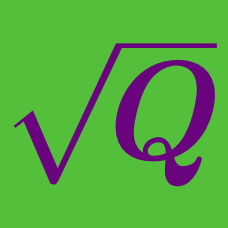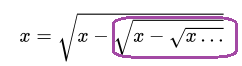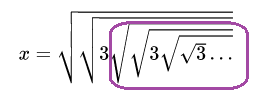Algebra

Radical Expressions and Equations

Challenge Quizzes

What's the correct statement about $x$?

$x = \sqrt{x - \sqrt{x - \sqrt{x \ldots } }}$

Hint: The expression marked in purple below is equivalent to $x$. Use substitution!$x = \sqrt{\sqrt{3\sqrt{\sqrt{3\sqrt{\sqrt{3} \ldots}}}}}$

Which of these is a solution for $x$?

Hint: The expression marked in purple below is equivalent to $x$. Use substitution!$x = \sqrt{2\sqrt{5\sqrt{2\sqrt{5 \ldots}}}}$

Which of these is a solution for $x$?

$x = \sqrt{2 \sqrt{x \sqrt{3 \sqrt{2 \sqrt{x \sqrt{3 \ldots } }}}}}$

What's the nonzero solution for $x$?

$x = \sqrt{a \sqrt{a \sqrt{a \sqrt{a \ldots }}}}$

If $a$ is nonzero, which of these does $x$ equal?

×